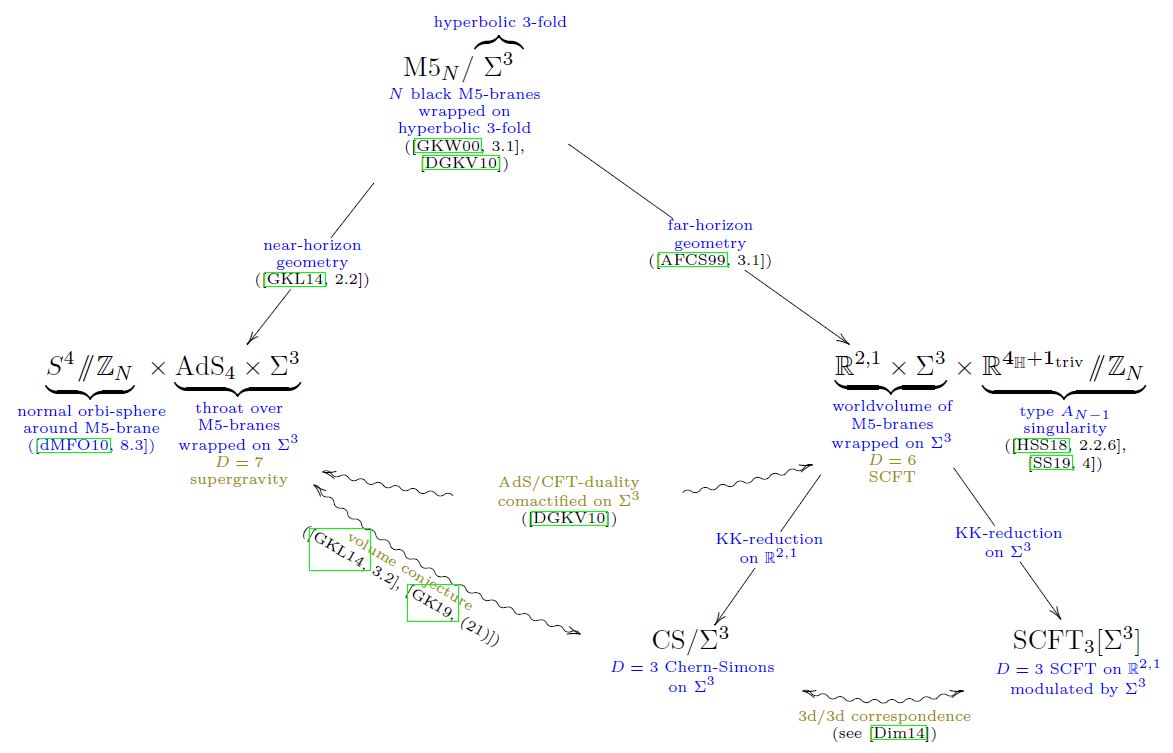# nLab volume conjecture

Contents

## Theorems

#### Functorial quantum field theory

functorial quantum field theory

# Contents

## Idea

The volume conjectures are a class of conjectures (slightly differing in generality and assumptions), saying that on a suitable (in particular: hyperbolic) 3-manifold $X$ a large N limit of SU(n)-Chern-Simons theory quantum observables ($N$-colored Jones polynomials or more generally RT-invariants, TV-invariants) is equal to the volume or complex volume of $X$.

For a few special cases of 3-manifolds there are explicit proofs of the volume conjecture(s). Besides this there is an abundance of numerical evidence for the volume conjectures, using computer algebra such as SnapPy (see also Zickert 07). In fact experimentation with these numerics is what has been driving the formulation of further variants of the volume conjecture.

Hence experimental mathematics strongly suggests that the volume conjectures are true. But a conceptual explanation (let alone proof) in terms of quantum field theory has remained open (Witten 14, bottom of p. 4).

But an explanation via duality in string theory, combining AdS/CFT duality with the 3d/3d correspondence for wrapped M5-branes, is argued for in Gang-Kim-Lee 14, 3.2, Gang-Kim 18 (21), see below.

### For $SU(2)$ on knot complements

The original volume conjecture (also “Kashaev’s conjecture”, due to Kashaev 95, and understood in terms of the $N$-colored Jones polynomial by Murakami-Murakami 01) states that the large N limit of the $N$-colored Jones polynomial (for gauge group SU(2)) of a knot $K$ gives the simplicial volume of its complement in the 3-sphere (for hyperbolic knots this is the volume of the complementary hyperbolic 3-manifold)

(1)$lim_{N \to \infty} \left( \frac{ 2 \pi log } {N} \left\vert V_N(K; q = e^{\frac{2 \pi i}{N}}) \right\vert \right) \;=\; vol(K).$

Here $V_N(K; q)$ is the ratio of the values of the $N$-colored Jones polynomial of $K$ and of the unknot

$V_N(K; q) = \frac{J_N(K; q)}{J_N(\bigcirc; q)}.$

The simplicial volume of a knot complement can be found via its unique torus decomposition into hyperbolic pieces and Seifert fibered pieces by a system of tori. The simplicial volume is then the sum of the hyperbolic volumes of the hyperbolic pieces of the decomposition.

If one omits the absolute value in (1) then the volume conjecture instead involves the complex volume (MMOTY 02, Conjecture 1.2).

### For $SU(2)$ on general 3-manifolds

More generally, volume conjectures state convergence of the Turaev-Viro invariants or Reshetikhin-Turaev invariants on general hyperbolic 3-manifolds to the volume or complex volume, respectively.

See (Chen-Yang 15)

### For $SU(n)$

Generalization from gauge group SU(2) to SU(n): Chen-Liu-Zhu 15

## Proof strategies

### As combined AdS/CFT + 3d/3d duality for wrapped M5-branes

In Gang-Kim-Lee 14b, 3.2, Gang-Kim 18 (21) it is argued that the volume conjecture for Chern-Simons theory on hyperbolic 3-manifolds $\Sigma^3$ is the combined statement of two dualities in string theory:

for the situation of M5-branes wrapped on $\Sigma^3$ (DGKV 10):### General

Original articles include

Generalization to Reshetikhin-Turaev construction on closed manifold, to the Turaev-Viro construction on manifolds with boundary, and to more general roots of unity than considered before is in

Generalization to SU(n):

Review includes

### Via string theory

#### General

Speculative discussion in terms of quantum field theory or string theory includes

A conceptual explanation of the volume conjecture via analytically continued Chern-Simons theory was proposed in

(but it seems that as a sketch or strategy for a rigorous proof, it didn’t catch on).

#### As AdS/CFT + 3d/3d duality for wrapped M5-branes

Suggestion that the statement of the volume conjecture is really AdS-CFT duality combined with the 3d-3d correspondence for M5-branes wrapped on hyperbolic 3-manifolds:

• Dongmin Gang, Nakwoo Kim, Sangmin Lee, Section 3.2 of: Holography of 3d-3d correspondence at Large $N$, JHEP 04 (2015) 091 (arXiv:1409.6206)

• Dongmin Gang, Nakwoo Kim, around (21) of: Large $N$ twisted partition functions in 3d-3d correspondence and Holography, Phys. Rev. D 99, 021901 (2019) (arXiv:1808.02797)